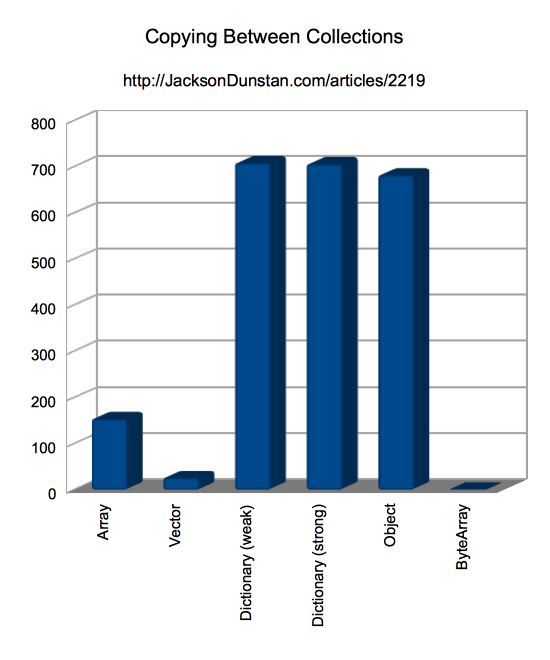Programming in AS3 invariably involves choosing between various collections: `Array`, `Vector`, `Dictionary`, `Object`, `ByteArray`, and so on. What if you need to quickly copy between them? Your choice of collection could result in a 450x slowdown in your app… or a 450x speedup!

The following test fills all collections with 10,000 integers and then copies 10,000 integers 1,000 times between two of the same collection types:

• `Array`
• `Vector`
• `Dictionary` (weak keys)
• `Dictionary` (strong keys)
• `Object`
• `ByteArray`

All of them except `ByteArray` use a simple `collection2[i] = collection[i]` loop. In the case of `ByteArray`, bytes are copied en masse using `ByteArray.readBytes` and a couple of `position` setters.

```package
{
import flash.text.TextField;
import flash.utils.getTimer;
import flash.utils.ByteArray;
import flash.utils.Dictionary;
import flash.display.Sprite;

public class CopyingBetweenCollections extends Sprite
{
public function CopyingBetweenCollections()
{
var SIZE:int = 10000;
var REPS:int = 1000;
var i:int;
var j:int;
var beforeTime:int;
var afterTime:int;

var array:Array = [];
var vector:Vector.<int> = new <int>[];
var dictionaryWeak:Dictionary = new Dictionary(true);
var dictionaryStrong:Dictionary = new Dictionary(false);
var object:Object = {};
var byteArray:ByteArray = new ByteArray();

var array2:Array = [];
var vector2:Vector.<int> = new <int>[];
var dictionaryWeak2:Dictionary = new Dictionary(false);
var dictionaryStrong2:Dictionary = new Dictionary(true);
var object2:Object = {};
var byteArray2:ByteArray = new ByteArray();

var tf:TextField = new TextField();
tf.width = stage.stageWidth;
tf.height = stage.stageHeight;
tf.text = "Collection,Time\n";

byteArray.length = SIZE;
for (i = 0; i < SIZE; ++i)
{
array[i] = i;
vector[i] = i;
dictionaryWeak[i] = i;
dictionaryStrong[i] = i;
object[i] = i;
byteArray[i] = i;

array2[i] = i;
vector2[i] = i;
dictionaryWeak2[i] = i;
dictionaryStrong2[i] = i;
object2[i] = i;
byteArray2[i] = i;
}

beforeTime = getTimer();
for (i = 0; i < REPS; ++i)
{
for (j = 0; j < SIZE; ++j)
{
array2[j] = array[j];
}
}
afterTime = getTimer();
tf.appendText("Array," + (afterTime-beforeTime) + "\n");

beforeTime = getTimer();
for (i = 0; i < REPS; ++i)
{
for (j = 0; j < SIZE; ++j)
{
vector2[j] = vector[j];
}
}
afterTime = getTimer();
tf.appendText("Vector," + (afterTime-beforeTime) + "\n");

beforeTime = getTimer();
for (i = 0; i < REPS; ++i)
{
for (j = 0; j < SIZE; ++j)
{
dictionaryWeak2[j] = dictionaryWeak[j];
}
}
afterTime = getTimer();
tf.appendText("Dictionary (weak)," + (afterTime-beforeTime) + "\n");

beforeTime = getTimer();
for (i = 0; i < REPS; ++i)
{
for (j = 0; j < SIZE; ++j)
{
dictionaryStrong2[j] = dictionaryStrong[j];
}
}
afterTime = getTimer();
tf.appendText("Dictionary (strong)," + (afterTime-beforeTime) + "\n");

beforeTime = getTimer();
for (i = 0; i < REPS; ++i)
{
for (j = 0; j < SIZE; ++j)
{
object2[j] = object[j];
}
}
afterTime = getTimer();
tf.appendText("Object," + (afterTime-beforeTime) + "\n");

beforeTime = getTimer();
for (i = 0; i < REPS; ++i)
{
byteArray.position = 0;
byteArray2.position = 0;
}
afterTime = getTimer();
tf.appendText("ByteArray," + (afterTime-beforeTime) + "\n");
}
}
}```

Launch the test

I ran this test in the following environment:

• Release version of Flash Player 11.7.700.169
• 2.3 Ghz Intel Core i7
• Mac OS X 10.8.3
• ASC 2.0 build 352231 (`-debug=false -verbose-stacktraces=false -inline`)

And here are the results I got:

Collection Time
Array 154
Vector 26
Dictionary (weak) 708
Dictionary (strong) 706
Object 682
ByteArray 1`Dictionary` (regardless of key strength) and `Object` are far and away the slowest collections to read and write from. Interestingly, `Object` is actually faster than `Dictionary` despite needing to translate `int` values to `String` keys. `Array` is about 3x faster than either of these, but still solidly in the “slow” camp compared to `Vector` and `ByteArray`.

`Vector` is about 6x faster than `Array` and 18x faster than `Dictionary` or `Object`, but it can’t touch `ByteArray` with its barely-measurable time of 1 millisecond. There were many cases while running the tests that it would register as 0 milliseconds, but the basic idea stands: it’s at least 25x faster than `Vector`, 150x faster than `Array`, and 450x faster than `Object` or `Dictionary`.

So if you need fast copying between collections, use a `ByteArray`. Second-best is a `Vector` if `ByteArray` offers too many downsides (e.g. it’s cumbersome).